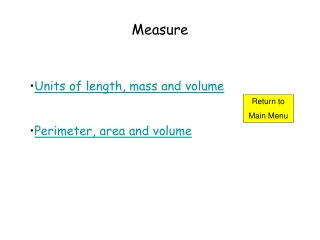DownloadDownload PresentationMeasure

# Measure

Download Presentation## Measure

- - - - - - - - - - - - - - - - - - - - - - - - - - - E N D - - - - - - - - - - - - - - - - - - - - - - - - - - -
##### Presentation Transcript

1. Measure • Units of length, mass and volume • Perimeter, area and volume Return to Main Menu

2. Measurement Measurements are made using the standard metric system. Units of length The standard unit of length is the metre (m). It is often more convenient to measure in decimal multiples of a metre such as: millimetres (mm), centimetres (cm) and kilometres (km).

3. Measurements Converting Units of Length

4. Examples Convert: • 2 m to cm. 2 m = 2 x 100 = 200 cm (b) 4 km to m. 4 km = 4 x 1000 = 4000 m (c) 34 cm to mm. 34 cm = 34 x 10 = 340 mm (d) 50 cm to m. 50 cm = 50 x 100 = 0.5 m

5. MeasurementUnits of Mass The standard unit of mass is the kilogram (kg). It is often more convenient to work in milligrams (mg) or grams (g). 1 kg = 1000g Kg g

6. Examples Convert: • 2 kg to g. 2 kg = 2 x 1000 = 2000 g (b) 4.6 kg to g. 4.6 kg = 4.6 x 1000 = 4600 g (c) 300g to kg. 300 g = 300 1000 = 0.3 kg

7. MeasurementUnits of Volume Volume is normally measured using m3, millilitres (ml) and litres (l). 1 ml = 1cm3 1 L = 1000ml L ml

8. End of Lesson Click to return back to main menu Click to give feedback on slide show

9. Perimeter The perimeter is the distance around a closed shape. Example Calculate the perimeter of the rectangle Perimeter = 6 + 6 + 2 + 2 2 cm = 16 cm 6 cm

10. height radius breadth base length Area Area is the amount of space enclosed within a 2D shape. Area formulae A = length x breadth

11. Volume Volume is the amount of space enclosed within a 3D container. height breadth length Volume = Length x breadth x height

12. End of Lesson Click to return back to main menu Click to give feedback on slide show